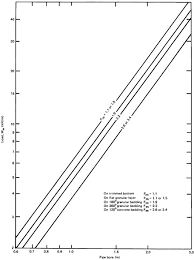## How to Calculate and Solve for Soil Load on Rigid Pipe | Irrigation Water RequirementThe image above represents soil load on rigid pipe.

To compute for soil load on rigid pipe, three essential parameters are needed and these parameters are Load Co-efficient for Ditch Conduits (Cd), Unit Weight of Fill Material (w) and Width of Ditch at Top of Conduit (Bd).

The formula for calculating Soil Load on Rigid Pipe:

Wc = CdwBd²

Where:

Wc = Soil Load on Rigid Pipe
Cd = Load Co-efficient for Ditch Conduits
w = Unit Weight of Fill Material
Bd = Width of Ditch at Top of Conduit

Let’s Solve an example;
Find the soil load on rigid pipe when the load co-efficient for ditch conduits is 16, the unit weight of fill material is 6 and width of ditch at top of conduit is 2.

This implies that;

Cd = Load Co-efficient for Ditch Conduits = 16
w = Unit Weight of Fill Material = 6
Bd = Width of Ditch at Top of Conduit = 2

Wc = CdwBd²
Wc = (16)(6)(2)²
Wc = (16)(6)(4)
Wc = 384

Therefore, the soil load on rigid pipe is 384.

Calculating for the Load Co-efficient for Ditch Conduits when the Soil Load on Rigid Pipe, the Unit Weight of Fill Material and the Width of Ditch at Top of Conduit is Given.

Cd = Wc / w x Bd2

Where;

Cd = Load Co-efficient for Ditch Conduits
Wc = Soil Load on Rigid Pipe
w = Unit Weight of Fill Material
Bd = Width of Ditch at Top of Conduit

Let’s Solve an example;
Find the load co-efficient for ditch conduits when the soil load on rigid pipe is 40, the unit weight of fill material is 4 and the Width of ditch at top of conduit is 2.

This implies that;

Wc = Soil Load on Rigid Pipe = 40
w = Unit Weight of Fill Material = 4
Bd = Width of Ditch at Top of Conduit = 2

Cd = Wc / w x Bd2
Cd = 40 / 4 x 22
Cd = 40 / 4 x 4
Cd = 40 / 16
Cd = 2.5

Therefore, the load co-efficient for ditch conduits is 2.5.